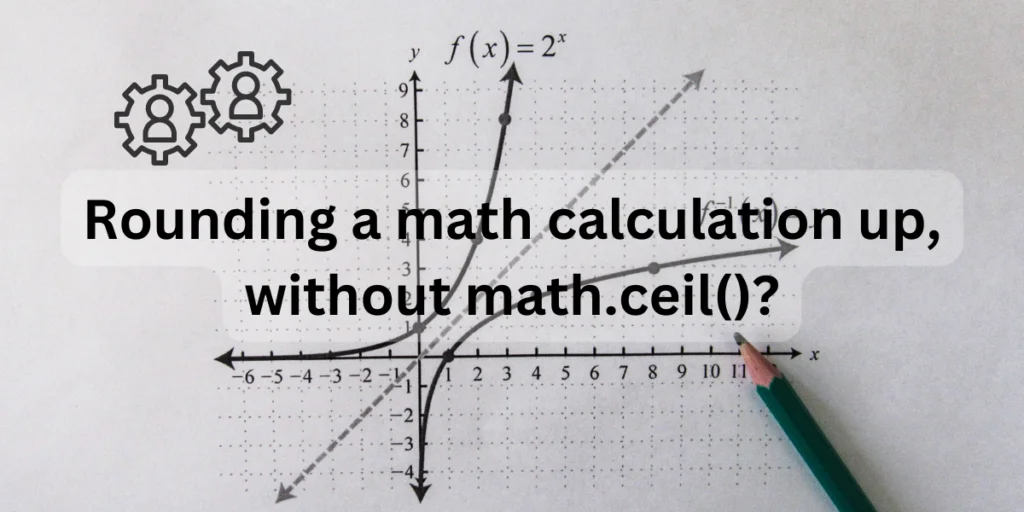# Round Up Numbers in Python Without math.ceil()The math module in Python provides a wide range of functionalities for mathematical functions. All of the functions present on your calculator have an equivalent in Python’s math module.

The math module provides all operations that can be carried out using real numbers. However, this module does not support operations on complex numbers. It provides all functionalities as per the C standard.

You can find the square root of numbers, remainders, use ceiling and floor functions as well using this module. It is very easy to install and has tons of substitutions for easy switching. You can also perform permutations and combinations with this module without having to write long functions for doing so!

The ceiling function is used to round up a number to it’s nearest whole number which is greater than the integer portion of that float. The math.ceil() function is normally used to perform the ceiling operation on floating type, but we can substitute it using the round() function or manually write a program with math.modf() to do so.

## Exploring the Python Math Module

The math module has an extensive collection of functions that can be used to perform different kinds of operations on real numbers. Although it is only limited to real numbers, the ease of using this function and it’s simplicity comes in handy always.

Some of the most important functions that can are present in this module are:

• math.sqrt(your_number): This function is used to calculate the square root of a given number.
• math.ceil(your_number): It works as the ceiling function for a particular number. It rounds your number up to the next integer greater than itself.
• math.floor(your_number): It works as the flooring function for a particular number. It rounds your number down to the integer less than itself.
• math.perm(n, m): It gives you the permutation for choosing m number of items from n.
• math.comb(n, m): It gives you the number of combinations in which m can be chosen from n.
• math.gcd(k, l): It finds the greatest common divisor or highest common factor of k and l.
• math.lcm(k, l): It finds the least common multiple of k and l.

You can install the math module in your system by opening your command prompt and running the following code:

```pip install python-math
```

## Using the round() Function as an Alternative to math.ceil()

Let’s see how are going to do this. First import the math module in your code. Next, take any float number as input, and use the math.ceil() function on it.

Then we are going to print this result.

After that, we are going to use the round() function and display it’s results. Both the answers will come out to be the same.

```#importing the math module
import math
#taking user input
inp=float(input("Enter a number= "))
#checking result using the ceiling function
print("The ceiling function result=",math.ceil(inp))
#using the round() function.
print("The round function result=",round(inp))
```

The output would be:

```Enter a number= 4.78
The ceiling function result= 5
The round function result= 5
```

Similar: Numpy round_ – Round an array to the given number of decimals.

## Using math.modf() as an Alternative to math.ceil()

In this method, we will use the math.modf() function which will separate the whole number portion from the fractional portion of a float type. We will add one to the whole number if the fractional portion is greater than or equal to 0.5 or else we will only print the whole number portion. This will be done using the if-else statement.

```#importing the math module
import math
#taking user input
inp=float(input("Enter a number= "))
#checking result using the ceiling function
print("The ceiling function result=",math.ceil(inp))
#using the modf function.
aftpoint, befpoint= math.modf(inp)
aftpoint=round(aftpoint,1)
if (aftpoint>=0.5):
print("The result is=",befpoint+1)
else:
print("The result is=",befpoint)
```

The output would be:

```Enter a number= 4.78
The ceiling function result= 5
The result is= 5.0
```